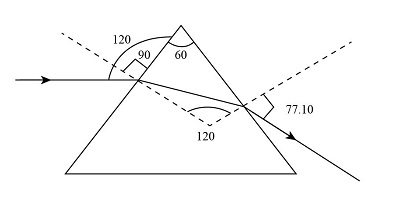# 1) An object is 24 cm away from a convex mirror. The image is 4 cm away from the mirror. Is the...

## Question:

1) An object is 24 cm away from a convex mirror. The image is 4 cm away from the mirror. Is the image real or virtual? What is the focal length of the mirror? If the object is 0.12 cm of height, what is the image size? What is the magnification?

2) A horizontal beam of light goes through a glass equilateral prism. Find its direction (angle to the horizon) after it goes through the prism. The index of refraction for glass is 1.5.

## Mirror Formula:

A ray diagram constructed for the convex mirror revealed that an image of the object is straight, decreased in size, and forms at back of the mirror. Mirror formula is a relationship between the focal length of a convex lens and the distance of the object and image from the lens.

Given data

• Distance of the object from the mirror is {eq}{d_o} = 24\;{\rm{cm}} = 0.24\;{\rm{m}} {/eq}
• Distance of the image from the mirror is {eq}{d_i} = 4\;{\rm{cm}} = 0.04\;{\rm{m}}{/eq}
• Height of the object is {eq}{h_o} = 0.12\;{\rm{cm}} {/eq}
• Redfractive index of the glass is {eq}n = 1.5 {/eq}
• Angle of prism is {eq}\theta = 60^\circ {/eq}
• First incident angle is {eq}{i_1} = 120^\circ - 90^\circ = 30^\circ {/eq}
• First refractive angle is {eq}{r_1} {/eq}
• Second incident angle (inside the prism) is {eq}{i_2} = 180^\circ - 120^\circ - {r_1} {/eq}
• Second refractive angle (inside the prism) is {eq}{r_2} {/eq}

The expression for the mirror formula is,

{eq}\dfrac{1}{f} = \dfrac{1}{u} + \dfrac{1}{v}......\left( 1 \right) {/eq}

The expression for the Snell?s law is,

{eq}\dfrac{{\sin i}}{{\sin r}} = \dfrac{{{n_2}}}{{{n_1}}}......\left( 2 \right) {/eq}

The expression for the magnification is,

{eq}m = - \dfrac{{{d_i}}}{{{d_o}}} = - \dfrac{{{h_i}}}{{{h_o}}}......\left( 3 \right) {/eq}

PART (a)

Substituting the given values in expression 1,

{eq}\begin{align*} \dfrac{1}{f} &= \dfrac{1}{{24}} + \dfrac{1}{4}\\ f &= 0.291\;{\rm{cm}} \end{align*} {/eq}

The nature of image is virtual.

Substituting the values in expression 3,

{eq}\begin{align*} m &= - \dfrac{4}{{24}}\\ m &= - 0.16 \end{align*} {/eq}

Further,

{eq}\begin{align*} m &= - \dfrac{{{h_i}}}{{0.12}}\\ {h_i} &= 0.02\;{\rm{cm}} \end{align*} {/eq}

PART (b)

The below diagram shows the refraction of light.Substituting the values in expression 2,

{eq}\begin{align*} \dfrac{{\sin \left( {30^\circ } \right)}}{{\sin {r_1}}} &= \dfrac{{1.5}}{1}\\ {r_1} &= 19.47^\circ \end{align*} {/eq}

Therefore, {eq}{i_2} = 40.53^\circ {/eq}

Substituting the values in expression 2,

{eq}\begin{align*} \dfrac{{\sin \left( {40.53^\circ } \right)}}{{\sin \;{r_2}}} &= \dfrac{1}{{1.5}}\\ {r_2} &= 77.10^\circ \end{align*} {/eq}

Therefore, the direction (angle to the horizon) after it goes through the prism is {eq}77.10^\circ - 30^\circ = 47.10^\circ {/eq}